# Pandas Fundamentals¶

This material is adapted from the Earth and Environmental Data Science, from Ryan Abernathey (Columbia University).

```import pandas as pd
import numpy as np

from matplotlib import pyplot as plt
# %config InlineBackend.figure_format = 'retina'
plt.ion()  # To trigger the interactive inline mode
```

## Pandas Data Structures: Series¶

A `Series` represents a one-dimensional array of data. The main difference between a `Series` and `numpy array` is that a `Series` has an index. The index contains the labels that we use to access the data.

There are many ways to create a Series. We will just show a few.

```names = ['Yasi', 'Debbie', 'Yasa']
values = [5., 4., 5.]
cyclones = pd.Series(values, index=names)
cyclones
```
```Yasi      5.0
Debbie    4.0
Yasa      5.0
dtype: float64
```

Series have built in plotting methods.

```cyclones.plot(kind='bar')
```
```<AxesSubplot:>
```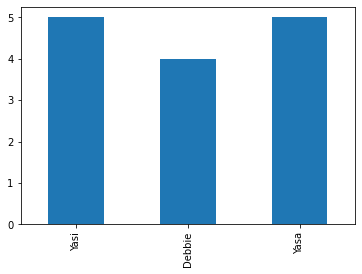Arithmetic operations and most numpy function can be applied to Series. An important point is that the Series keep their index during such operations.

```np.log(cyclones) / cyclones**2
```
```Yasi      0.064378
Debbie    0.086643
Yasa      0.064378
dtype: float64
```

We can access the underlying index object if we need to:

```cyclones.index
```
```Index(['Yasi', 'Debbie', 'Yasa'], dtype='object')
```

### Indexing¶

We can get values back out using the index via the `.loc` attribute

```cyclones.loc['Debbie']
```
```4.0
```

Or by raw position using `.iloc`

```cyclones.iloc
```
```4.0
```

We can pass a list or array to loc to get multiple rows back:

```cyclones.loc[['Debbie', 'Yasa']]
```
```Debbie    4.0
Yasa      5.0
dtype: float64
```

And we can even use slice notation

```cyclones.loc['Debbie':'Yasa']
```
```Debbie    4.0
Yasa      5.0
dtype: float64
```
```cyclones.iloc[:2]
```
```Yasi      5.0
Debbie    4.0
dtype: float64
```

If we need to, we can always get the raw data back out as well

```cyclones.values # a numpy array
```
```array([5., 4., 5.])
```
```cyclones.index # a pandas Index object
```
```Index(['Yasi', 'Debbie', 'Yasa'], dtype='object')
```

## Pandas Data Structures: DataFrame¶

There is a lot more to Series, but they are limit to a single “column”. A more useful Pandas data structure is the DataFrame. A DataFrame is basically a bunch of series that share the same index. It’s a lot like a table in a spreadsheet.

Below we create a DataFrame.

```# first we create a dictionary
data = {'category': [5, 4, 5],
'windspeed': [250., 215., 260.],
'fatalities': [1, 14, 4]}
df = pd.DataFrame(data, index=['Yasi', 'Debbie', 'Yasa'])
df
```
category windspeed fatalities
Yasi 5 250.0 1
Debbie 4 215.0 14
Yasa 5 260.0 4

Pandas handles missing data very elegantly, keeping track of it through all calculations.

```df.info()
```
```<class 'pandas.core.frame.DataFrame'>
Index: 3 entries, Yasi to Yasa
Data columns (total 3 columns):
#   Column      Non-Null Count  Dtype
---  ------      --------------  -----
0   category    3 non-null      int64
1   windspeed   3 non-null      float64
2   fatalities  3 non-null      int64
dtypes: float64(1), int64(2)
memory usage: 96.0+ bytes
```

A wide range of statistical functions are available on both Series and DataFrames.

```df.min()
```
```category        4.0
windspeed     215.0
fatalities      1.0
dtype: float64
```
```df.mean()
```
```category        4.666667
windspeed     241.666667
fatalities      6.333333
dtype: float64
```
```df.std()
```
```category       0.577350
windspeed     23.629078
fatalities     6.806859
dtype: float64
```
```df.describe()
```
category windspeed fatalities
count 3.000000 3.000000 3.000000
mean 4.666667 241.666667 6.333333
std 0.577350 23.629078 6.806859
min 4.000000 215.000000 1.000000
25% 4.500000 232.500000 2.500000
50% 5.000000 250.000000 4.000000
75% 5.000000 255.000000 9.000000
max 5.000000 260.000000 14.000000

We can get a single column as a Series using python’s getitem syntax on the DataFrame object.

```df['windspeed']
```
```Yasi      250.0
Debbie    215.0
Yasa      260.0
Name: windspeed, dtype: float64
```

…or using attribute syntax.

```df.windspeed
```
```Yasi      250.0
Debbie    215.0
Yasa      260.0
Name: windspeed, dtype: float64
```

Indexing works very similar to series

```df.loc['Debbie']
```
```category        4.0
windspeed     215.0
fatalities     14.0
Name: Debbie, dtype: float64
```
```df.iloc
```
```category        4.0
windspeed     215.0
fatalities     14.0
Name: Debbie, dtype: float64
```

But we can also specify the column we want to access

```df.loc['Debbie', 'fatalities']
```
```14
```
```df.iloc[:2, 2]
```
```Yasi       1
Debbie    14
Name: fatalities, dtype: int64
```

If we make a calculation using columns from the DataFrame, it will keep the same index:

```df.windspeed / df.category
```
```Yasi      50.00
Debbie    53.75
Yasa      52.00
dtype: float64
```

Which we can easily add as another column to the DataFrame:

```df['ratio'] = df.windspeed / df.category
df
```
category windspeed fatalities ratio
Yasi 5 250.0 1 50.00
Debbie 4 215.0 14 53.75
Yasa 5 260.0 4 52.00

## Merging Data¶

Pandas supports a wide range of methods for merging different datasets. These are described extensively in the documentation. Here we just give a few examples.

```cyclone = pd.Series(['\$246.7 M', None, '\$2.73 B', '\$3.6 B'],
index=['Yasa', 'Tino', 'Debbie', 'Yasi'],
name='cost')
cyclone
```
```Yasa      \$246.7 M
Tino          None
Debbie     \$2.73 B
Yasi        \$3.6 B
Name: cost, dtype: object
```
```# returns a new DataFrame
df.join(cyclone)
```
category windspeed fatalities ratio cost
Yasi 5 250.0 1 50.00 \$3.6 B
Debbie 4 215.0 14 53.75 \$2.73 B
Yasa 5 260.0 4 52.00 \$246.7 M
```# returns a new DataFrame
df.join(cyclone, how='right')
```
category windspeed fatalities ratio cost
Yasa 5.0 260.0 4.0 52.00 \$246.7 M
Tino NaN NaN NaN NaN None
Debbie 4.0 215.0 14.0 53.75 \$2.73 B
Yasi 5.0 250.0 1.0 50.00 \$3.6 B
```# returns a new DataFrame
cyc = df.reindex(['Yasa', 'Tino', 'Debbie', 'Yasi', 'Tracy'])
cyc
```
category windspeed fatalities ratio
Yasa 5.0 260.0 4.0 52.00
Tino NaN NaN NaN NaN
Debbie 4.0 215.0 14.0 53.75
Yasi 5.0 250.0 1.0 50.00
Tracy NaN NaN NaN NaN

We can also index using a boolean series. This is very useful

```huge = df[df.category > 4]
huge
```
category windspeed fatalities ratio
Yasi 5 250.0 1 50.0
Yasa 5 260.0 4 52.0
```df['is_huge'] = df.category > 4
df
```
category windspeed fatalities ratio is_huge
Yasi 5 250.0 1 50.00 True
Debbie 4 215.0 14 53.75 False
Yasa 5 260.0 4 52.00 True

### Modifying Values¶

We often want to modify values in a dataframe based on some rule. To modify values, we need to use `.loc` or `.iloc`

```df.loc['Debbie', 'windspeed'] = 285
df.loc['Yasa', 'category'] += 1
df
```
category windspeed fatalities ratio is_huge
Yasi 5 250.0 1 50.00 True
Debbie 4 285.0 14 53.75 False
Yasa 6 260.0 4 52.00 True

## Plotting¶

DataFrames have all kinds of useful plotting built in.

```df.plot(kind='scatter', x='category', y='windspeed', grid=True)
```
```<AxesSubplot:xlabel='category', ylabel='windspeed'>
```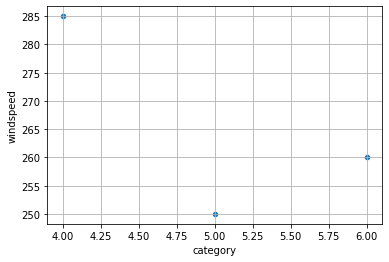```df.plot(kind='bar')
```
```<AxesSubplot:>
```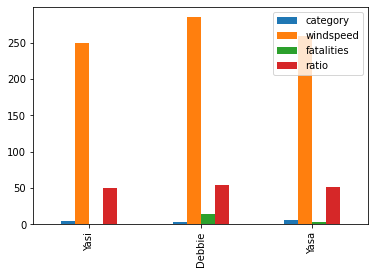## Time Indexes¶

Indexes are very powerful. They are a big part of why Pandas is so useful. There are different indices for different types of data. Time Indexes are especially great!

```two_years = pd.date_range(start='2014-01-01', end='2016-01-01', freq='D')
timeseries = pd.Series(np.sin(2 *np.pi *two_years.dayofyear / 365),
index=two_years)
timeseries.plot()
```
```<AxesSubplot:>
```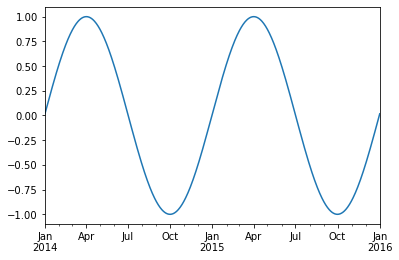We can use python’s slicing notation inside `.loc` to select a date range.

```timeseries.loc['2015-01-01':'2015-07-01'].plot()
```
```<AxesSubplot:>
```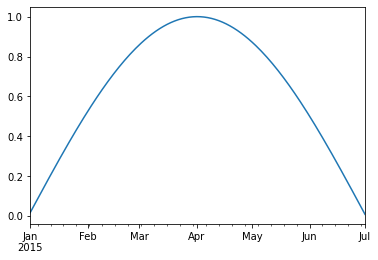The TimeIndex object has lots of useful attributes

```timeseries.index.month
```
```Int64Index([ 1,  1,  1,  1,  1,  1,  1,  1,  1,  1,
...
12, 12, 12, 12, 12, 12, 12, 12, 12,  1],
dtype='int64', length=731)
```
```timeseries.index.day
```
```Int64Index([ 1,  2,  3,  4,  5,  6,  7,  8,  9, 10,
...
23, 24, 25, 26, 27, 28, 29, 30, 31,  1],
dtype='int64', length=731)
```

In this example, we will use the eReefs Extraction tool from AIMS and look at Davies Reef which is located at 18.8S/147.6E.

The CSV file was downloaded from the AIMS extraction tool with the following settings:

• Data collection: GBR1 Hydro (Version 2)

• Variables: Eastward wind speed (wspeed_u), Northward wind speed (wspeed_v), Northward current (v), Eastward current (u)

• Date range: 2010-2021

• Time step: hourly Depths: -2.35 m

Once the extraction request was submitted the dataset was created after an one hour of processing the data was available for download from Extraction request: Example dataset: Wind-vs-Current at Davies and Myrmidon Reefs (2019).

After download we have a file called `DaviesReef_timeseries.csv`.

Tip

To read it into pandas, we will use the read_csv function. This function is incredibly complex and powerful. You can use it to extract data from almost any text file. However, you need to understand how to use its various options.

With no options, this is what we get.

```df = pd.read_csv('examples/DaviesReef_timeseries.csv')
```
date current wind salinity temperature
0 2010-09-01 0.18839505 7.481102 35.47361 25.17717
1 2010-09-02 0.28618774 4.9959917 35.48525 25.26...
2 2010-09-03 0.29367417 6.024843 35.462696 25.20...
3 2010-09-04 0.28546846 5.7049136 35.44163 25.38...
4 2010-09-05 0.34016508 4.3658934 35.41293 25.7196

Pandas failed to identify the different columns. This is because it was expecting standard CSV (comma-separated values) file. In our file, instead, the values are separated by whitespace. And not a single whilespace–the amount of whitespace between values varies. We can tell pandas this using the `sep` keyword.

```df = pd.read_csv('examples/DaviesReef_timeseries.csv', sep='\s+')
```
date current wind salinity temperature
0 2010-09-01 0.188395 7.481102 35.473610 25.177170
1 2010-09-02 0.286188 4.995992 35.485250 25.262072
2 2010-09-03 0.293674 6.024843 35.462696 25.201515
3 2010-09-04 0.285468 5.704914 35.441630 25.385157
4 2010-09-05 0.340165 4.365893 35.412930 25.719600

Great! It worked.

Often missing values are set either to -9999 or -99. Let’s tell this to pandas by assigning nan to these specific values.

```df = pd.read_csv('examples/DaviesReef_timeseries.csv', sep='\s+', na_values=[-9999.0, -99.0])
```
date current wind salinity temperature
0 2010-09-01 0.188395 7.481102 35.473610 25.177170
1 2010-09-02 0.286188 4.995992 35.485250 25.262072
2 2010-09-03 0.293674 6.024843 35.462696 25.201515
3 2010-09-04 0.285468 5.704914 35.441630 25.385157
4 2010-09-05 0.340165 4.365893 35.412930 25.719600

Great. The missing data is now represented by `NaN`.

What data types did pandas infer?

```df.info()
```
```<class 'pandas.core.frame.DataFrame'>
RangeIndex: 3815 entries, 0 to 3814
Data columns (total 5 columns):
#   Column       Non-Null Count  Dtype
---  ------       --------------  -----
0   date         3815 non-null   object
1   current      3815 non-null   float64
2   wind         3815 non-null   float64
3   salinity     3815 non-null   float64
4   temperature  3815 non-null   float64
dtypes: float64(4), object(1)
memory usage: 149.1+ KB
```

One problem here is that Pandas did not recognize the `date` column as a date. Let’s help it.

```df = pd.read_csv('examples/DaviesReef_timeseries.csv', sep='\s+',
na_values=[-9999.0, -99.0],
parse_dates=['date'])
df.info()
```
```<class 'pandas.core.frame.DataFrame'>
RangeIndex: 3815 entries, 0 to 3814
Data columns (total 5 columns):
#   Column       Non-Null Count  Dtype
---  ------       --------------  -----
0   date         3815 non-null   datetime64[ns]
1   current      3815 non-null   float64
2   wind         3815 non-null   float64
3   salinity     3815 non-null   float64
4   temperature  3815 non-null   float64
dtypes: datetime64[ns](1), float64(4)
memory usage: 149.1 KB
```

It worked! Finally, let’s tell pandas to use the date column as the index.

```df = df.set_index('date')
```
current wind salinity temperature
date
2010-09-01 0.188395 7.481102 35.473610 25.177170
2010-09-02 0.286188 4.995992 35.485250 25.262072
2010-09-03 0.293674 6.024843 35.462696 25.201515
2010-09-04 0.285468 5.704914 35.441630 25.385157
2010-09-05 0.340165 4.365893 35.412930 25.719600

We can now access values by time:

```df.loc['2014-08-07']
```
```current         0.381858
wind           11.198019
salinity       35.307660
temperature    23.866120
Name: 2014-08-07 00:00:00, dtype: float64
```

Or use slicing to get a range:

```df2014 = df.loc['2014-01-01':'2014-12-31']
df2016 = df.loc['2016-01-01':'2016-12-31']
df2018 = df.loc['2018-01-01':'2018-12-31']
df2020 = df.loc['2020-01-01':'2020-12-31']
df2014
```
current wind salinity temperature
date
2014-01-01 0.400576 5.939211 35.446510 28.987250
2014-01-02 0.382042 3.748479 35.437440 29.117306
2014-01-03 0.396745 4.321397 35.470460 29.362583
2014-01-04 0.438115 5.537916 35.474777 29.393948
2014-01-05 0.440387 5.804630 35.441086 29.072742
... ... ... ... ...
2014-12-27 0.229368 5.385712 35.412727 28.865137
2014-12-28 0.205974 3.610898 35.416306 29.263445
2014-12-29 0.303206 3.092528 35.432480 29.667050
2014-12-30 0.254966 2.479212 35.479332 30.116217
2014-12-31 0.285224 2.497501 35.500930 30.287010

365 rows × 4 columns

### Quick Statistics¶

```df.describe()
```
current wind salinity temperature
count 3815.000000 3815.000000 3815.000000 3815.000000
mean 0.265826 6.708479 35.182442 26.610127
std 0.084196 2.643628 0.292870 1.936466
min 0.061780 1.245048 32.956253 22.933332
25% 0.209833 4.613128 35.042687 24.853140
50% 0.256591 6.528472 35.263058 26.700006
75% 0.308909 8.581003 35.375860 28.180752
max 0.817050 18.900612 35.763687 31.598990

### Plotting Values¶

We can now quickly make plots of the data

```fig, ax = plt.subplots(ncols=2, nrows=2, figsize=(14,14))

df2014.iloc[:, :].boxplot(ax=ax[0,0])
df2016.iloc[:, :].boxplot(ax=ax[0,1])
df2018.iloc[:, :].boxplot(ax=ax[1,0])
df2020.iloc[:, :].boxplot(ax=ax[1,1])
```
```<AxesSubplot:>
```Pandas is very “time aware”:

```df.temperature.plot()
```
```<AxesSubplot:xlabel='date'>
```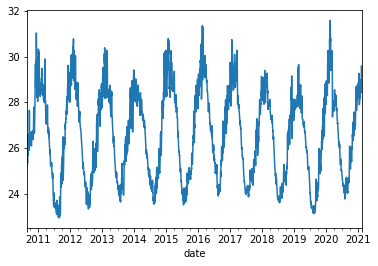Note: we could also manually create an axis and plot into it.

```fig, ax = plt.subplots()
df2018.temperature.plot(ax=ax)
```
```Text(0.5, 1.0, 'Pandas Made This!')
``````df2020[['temperature', 'wind', 'salinity']].plot()
```
```<AxesSubplot:xlabel='date'>
```### Resampling¶

Since pandas understands time, we can use it to do resampling.

```# monthly reampler object
rs_obj = df.resample('MS')
rs_obj
```
```<pandas.core.resample.DatetimeIndexResampler object at 0x7fb2ce581d30>
```
```rs_obj.mean()
```
current wind salinity temperature
date
2010-09-01 0.281347 5.869099 35.365671 26.017173
2010-10-01 0.283938 8.118046 35.368278 26.372507
2010-11-01 0.268329 7.007014 35.384552 26.872662
2010-12-01 0.342128 5.501357 35.019846 29.165949
2011-01-01 0.306163 5.960649 34.620039 29.236425
... ... ... ... ...
2020-10-01 0.257407 6.045590 35.242590 25.873325
2020-11-01 0.268678 6.381081 35.325458 27.001825
2020-12-01 0.288298 5.469824 35.251699 28.221937
2021-01-01 0.289114 7.052687 34.391954 28.522526
2021-02-01 0.236967 3.992934 34.692678 29.264261

126 rows × 4 columns

We can chain all of that together

```df_mm = df.resample('MS').mean()
df_mm[['temperature', 'wind', 'salinity']].plot()
```
```<AxesSubplot:xlabel='date'>
```Next notebook, we will dig deeper into resampling, rolling means, and grouping operations (groupby).